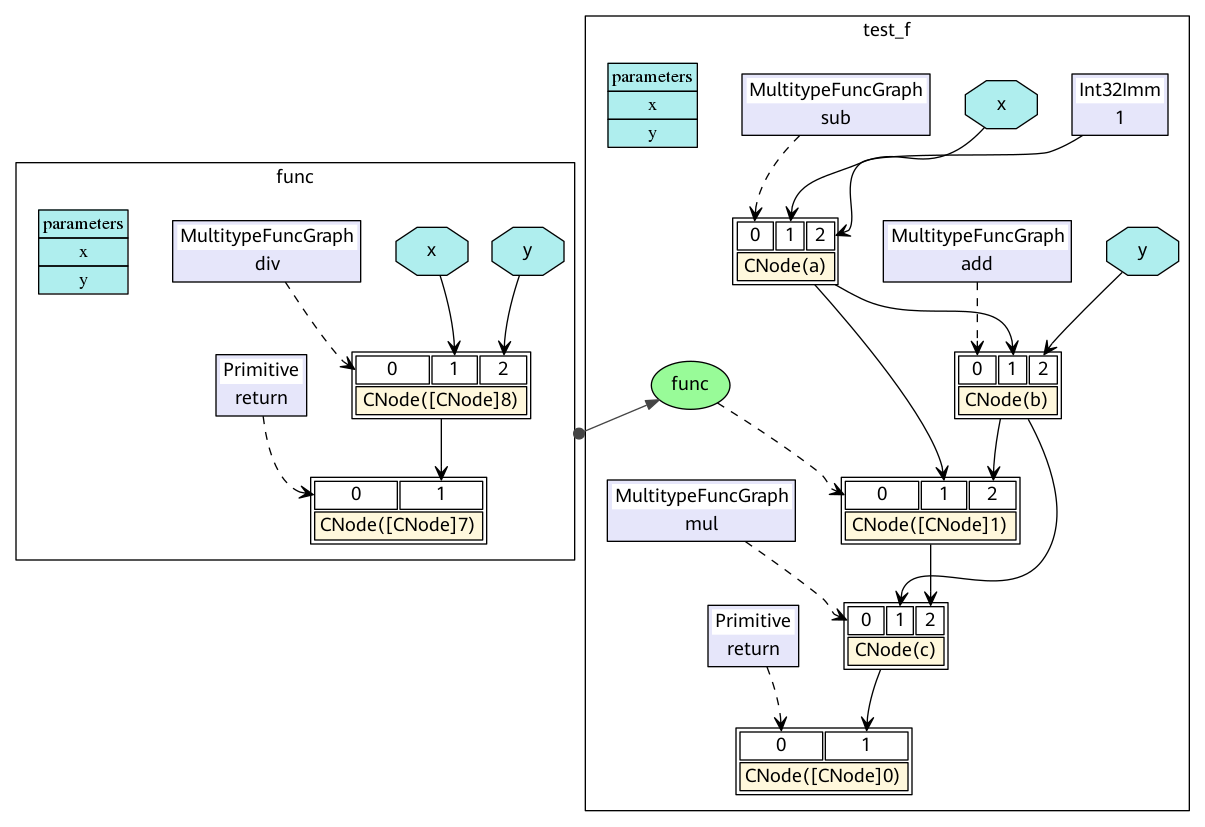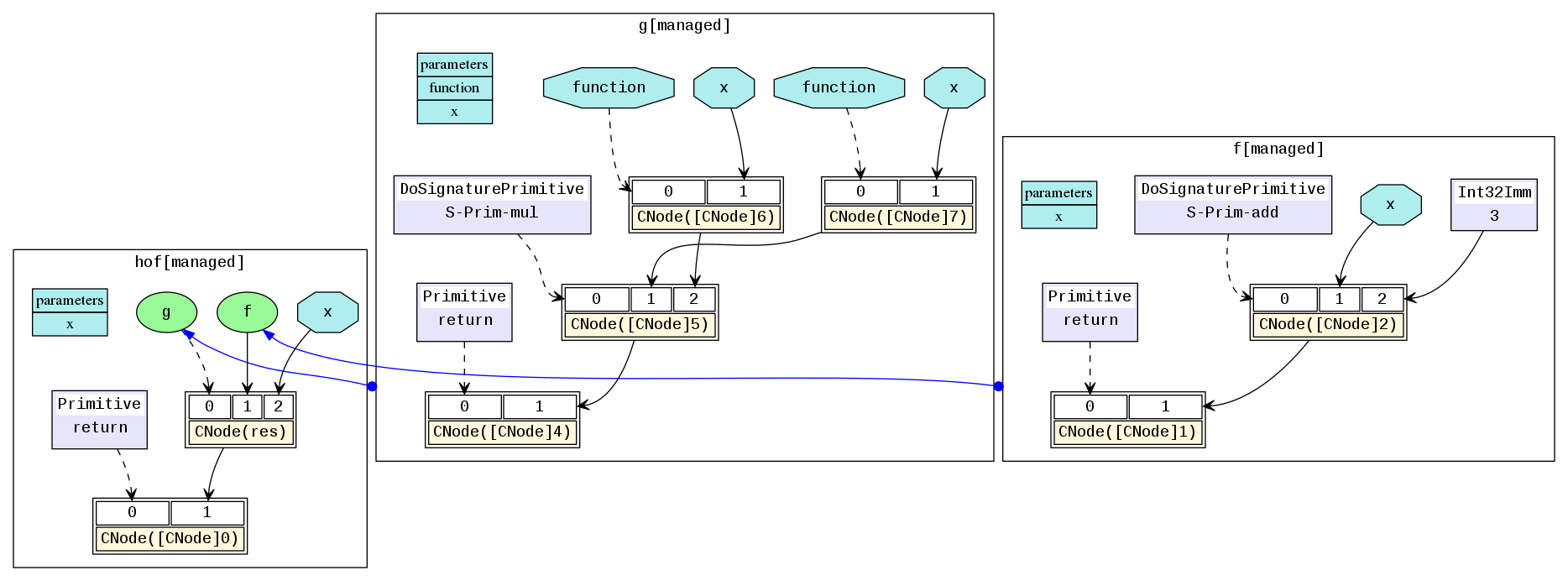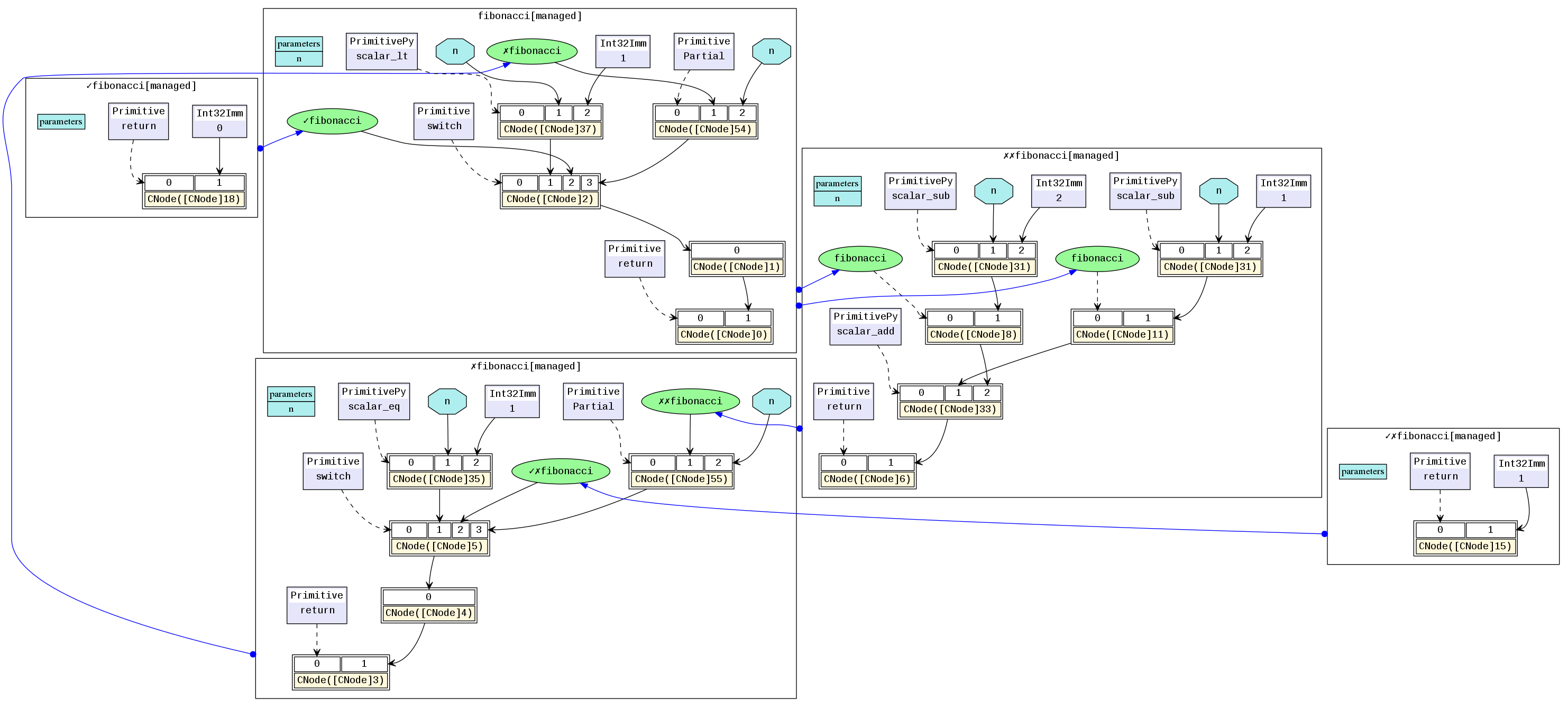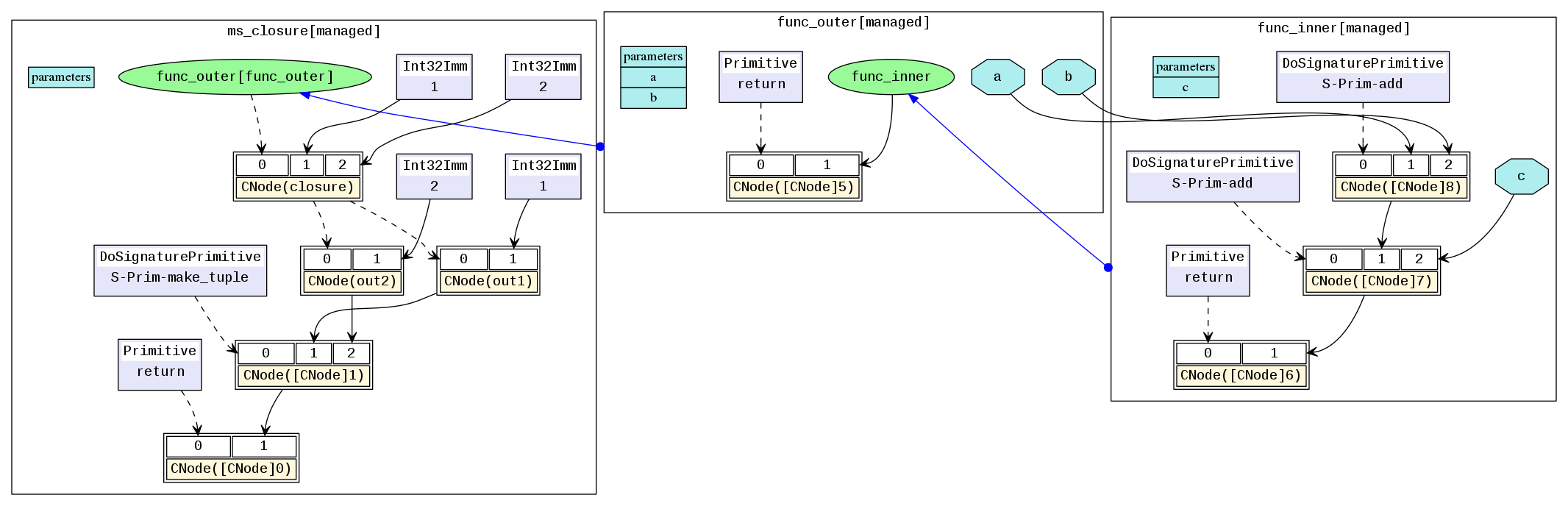# 中间表示MindIR## 简介

MindIR是一种基于图表示的函数式IR，其最核心的目的是服务于自动微分变换。自动微分采用的是基于函数式编程框架的变换方法，因此IR采用了接近于ANF函数式的语义。此外，借鉴Sea of Nodes和Thorin的优秀设计，采用了一种基于显性依赖图的表示方式。关于ANF-IR的具体介绍，可以参考MindSpore IR文法定义

• ir后缀结尾的IR文件：一种比较直观易懂的以文本格式描述模型结构的文件，可以直接用文本编辑软件查看。

• 通过设置环境变量`export MS_DEV_SAVE_GRAPTHS_SORT_MODE=1`可以生成的ir后缀结尾的IR文件：格式跟默认的ir文件基本相同，但是生成的异序ir文件的图的生成与打印顺序和默认ir文件不同。

• dot后缀结尾的IR文件：描述了不同节点间的拓扑关系，可以用graphviz将此文件作为输入生成图片，方便用户直观地查看模型结构。对于算子比较多的模型，推荐使用可视化组件MindInsight对计算图进行可视化。

## 文法定义

ANF是函数式编程中常用且简洁的中间表示，其文法定义如下所示：

```<aexp> ::= NUMBER | STRING | VAR | BOOLEAN | PRIMOP
|  (lambda (VAR …) <exp>)
<cexp> ::= (<aexp> <aexp> …)
|  (if <aexp> <exp> <exp>)
<exp> ::= (let ([VAR <cexp>]) <exp>) | <cexp> | <aexp>
```

ANF中表达式分为原子表达式（aexp）和复合表达式（cexp），原子表达式表示一个常数值或一个变量或一个匿名函数；复合表达式由多个原子表达式复合组成，表示一个匿名函数或原语函数调用，组合的第一个输入是调用的函数，其余输入是调用的参数。

MindIR文法继承于ANF，其定义如下所示：

```<ANode> ::= <ValueNode> | <ParameterNode>
<ParameterNode> ::= Parameter
<ValueNode> ::= Scalar | Named | Tensor | Type | Shape
| Primitive | MetaFuncGraph | FuncGraph
<CNode> ::= (<AnfNode> …)
<AnfNode> ::= <CNode> | <ANode>
```

MindIR中的ANode对应于ANF的原子表达式，ANode有两个子类分别为ValueNode和ParameterNode。ValueNode表示常数节点，可承载一个常数值（标量、符号、张量、类型、维度等），也可以是一个原语函数（Primitive）或一个元函数（MetaFuncGraph）或一个普通函数（FuncGraph），因为在函数式编程中函数定义本身也是一个值。ParameterNode是参数节点，表示函数的形参。

MindIR中CNode对应于ANF的复合表达式，表示一次函数调用。

## 示例

```def func(x, y):
return x / y

@ms.jit
def test_f(x, y):
a = x - 1
b = a + y
c = b * func(a, b)
return c
```

```lambda (x, y)
let a = x - 1 in
let b = a + y in
let func = lambda (x, y)
let ret = x / y in
ret end in
let %1 = func(a, b) in
let c = b * %1 in
c end
```## 函数式语义

MindIR较传统计算图的一个重要特性是不仅可以表达算子之间的数据依赖，还可以表达丰富的函数式语义。

### 高阶函数

```@ms.jit
def hof(x):
def f(x):
return x + 3
def g(function, x):
return function(x) * function(x)
res = g(f, x)
return res
```### 控制流

```@ms.jit
def fibonacci(n):
if(n < 1):
return 0
elif(n == 1):
return 1
else:
return fibonacci(n-1) + fibonacci(n-2)
```### 自由变量和闭包

```@ms.jit
def func_outer(a, b):
def func_inner(c):
return a + b + c
return func_inner

@ms.jit
def ms_closure():
closure = func_outer(1, 2)
out1 = closure(1)
out2 = closure(2)
return out1, out2
```## 参考文献

 C. Click and M. Paleczny. A simple graph-based intermediate representation. SIGPLAN Not., 30:35–49, March 1995.

 Roland Leißa, Marcel Köster, and Sebastian Hack. A graph-based higher-order intermediate representation. In Proceedings of the 13th Annual IEEE/ACM International Symposium on Code Generation and Optimization, pages 202–212. IEEE Computer Society, 2015.